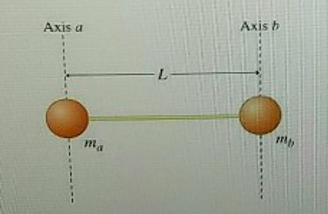# Problem: Ball a, of mass ma, is connected to ball b, of mass mb, by a massless rod of length L. The two vertical dashed lines in the figure, one through each ball represent two different axes of rotation, axes a and b. These axes are parallel to each other and perpendicular to the rod. The moment of inertia of the two-mass system about axis a is Ia, and the moment of inertia of the system about axis b to Ib. It is observed that the ratio of Ia to Ib is equal to 3.Ia/Ib = 3Assume that both balls are pointlike: that is, neither has any moment of inertia about its own center of mass.Part A. Find the ratio of the masses of the two balls. Express your answer numerically.Part B. Find da, the distance from the ball a to the system’s center of mass.Express your answer in terms of L, the length of the rod.

###### FREE Expert Solution

Moment of inertia:

$\overline{){\mathbf{I}}{\mathbf{=}}{\mathbf{\Sigma }}{\mathbf{m}}{{\mathbf{r}}}^{{\mathbf{2}}}}$

We've been given:

$\overline{)\frac{{\mathbf{I}}_{\mathbf{a}}}{{\mathbf{I}}_{\mathbf{b}}}{\mathbf{=}}{\mathbf{3}}}$

Let's take the axis to be at a.

Now we'll have:

$\begin{array}{rcl}{\mathbf{I}}_{\mathbf{a}}& \mathbf{=}& {\mathbf{m}}_{\mathbf{a}}{{\mathbf{r}}_{\mathbf{a}}}^{\mathbf{2}}\mathbf{+}{\mathbf{m}}_{\mathbf{b}}{{\mathbf{r}}_{\mathbf{b}}}^{\mathbf{2}}\\ & \mathbf{=}& {\mathbf{m}}_{\mathbf{a}}{\mathbf{\left(}\mathbf{0}\mathbf{\right)}}^{\mathbf{2}}\mathbf{+}{\mathbf{m}}_{\mathbf{b}}{\mathbf{\left(}\mathbf{L}\mathbf{\right)}}^{\mathbf{2}}\\ & \mathbf{=}& \mathbf{0}\mathbf{+}{\mathbf{m}}_{\mathbf{b}}{\mathbf{L}}^{\mathbf{2}}\end{array}$

95% (135 ratings)###### Problem Details

Ball a, of mass ma, is connected to ball b, of mass mb, by a massless rod of length L. The two vertical dashed lines in the figure, one through each ball represent two different axes of rotation, axes a and b. These axes are parallel to each other and perpendicular to the rod. The moment of inertia of the two-mass system about axis a is Ia, and the moment of inertia of the system about axis b to Ib. It is observed that the ratio of Ia to Ib is equal to 3.
Ia/Ib = 3
Assume that both balls are pointlike: that is, neither has any moment of inertia about its own center of mass.Part A. Find the ratio of the masses of the two balls. Express your answer numerically.

Part B. Find da, the distance from the ball a to the system’s center of mass.
Express your answer in terms of L, the length of the rod.